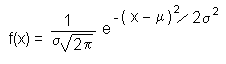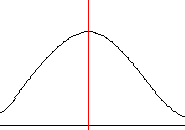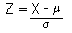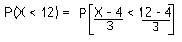## Title

Normal Distribution
Quick revise

A continuous random variable X follows a normal distribution if it has the following probability density function (p.d.f.):The parameters of the distribution are m and s2, where m is the mean (expectation) of the distribution and s2 is the variance. We write X ~ N(m, s2) to mean that the random variable X has a normal distribution with parameters m and s2.

The normal distribution is symmetrical about its mean:The Standard Normal Distribution

If Z ~ N(0, 1), then Z is said to follow a standard normal distribution.

P(Z < z) is known as the cumulative distribution function of the random variable Z. For the standard normal distribution, this is usually denoted by F(z).

Normally, you would work out the c.d.f. by doing some integration. However, it is impossible to do this for the normal distribution and so results have to be looked up in statistical tables.

Example

Find P(-1.96 < Z < 1.96), where Z ~ N(0, 1).

This is equal to P(Z < 1.96) - P(Z < -1.96)
= F(1.96) - F(-1.96) = F(1.96) - (1 - F(1.96)) = 2 F(1.96) - 1

Now we need to look in a table to find out what F(1.96) is.

If you look in statistical tables for the standard normal distribution, you should be able to find a line:

 z 0 1 2 3 4 5 6 7 8 9 1.9 0.9713 0.9719 0.9726 0.9732 0.9738 0.9744 0.975 0.9756 0.9761 0.9767

To find F(1.96), read across the 1.9 line until you get to 6 (for 1.96). So F(1.96) = 0.975 .

Hence P(-1.96 < Z < 1.96) = 2 × 0.975 - 1 = 0.95 .

This result says that the central 95% of the distribution lies between -1.96 and 1.96 .

Some Identities

• F(-z) = P(Z < -z) = 1 - F(z)

• P(Z > z) = 1 - F(z)

• P(a < Z < b) = F(b) - F(a)

Standardising

Now, the mean and variance of the normal distribution can be any value and so clearly there can't be a statistical table for each one. Instead, we convert to the standard normal distribution- we can also use statistical tables for the standard normal distribution to find the c.d.f. of any normal distribution.

We use the following trick:

If X ~ N(m, s2), then put:It turns out that Z ~ N(0, 1). Note that it is s and not s2 on the denominator!

Example

If X ~ N(4, 9), find P(X<12).

First we "standardise". The mean is 4 and s is 3 (the square root of 9).(subtracting 4 and dividing each side of the inequality by 3)

= P(Z < 8/3)

= F(8/3) = F(2.67) = 0.9962

Rate: